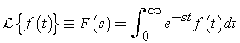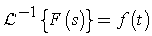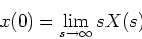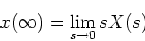### Electric Circuit and Electron Device - TRANSIENT RESONANCE IN RLC CIRCUITS (Unit 2)–Lecture Notes

Anna University

Electric Circuit and Electron Device

Subject Code : EC2151

Subject Name : Electric Circuit and Electron Device

Year : 1st yr

Semester : 2nd Sem

Department : CSE & ECE

UNIT-II

TRANSIENT RESONANCE IN RLC CIRCUITS

Transient State:

If a network contains energy storage elements, with change in excitation, the current and voltages change from one state to another state is called transient state. The behavior of the voltage or current when it is changed from one state to another state is called transient state.

Transient Time:

The time taken for the circuit to change from one steady state to another steady state is called the transient time.

Natural response:

If we consider a circuit containing storage elements which are independent of sources, the response depends upon the nature of the circuit, it is called natural response.

Transient response:

The storage elements deliver their energy to the resistances, hence the response changes with time, gets saturated after sometime, and is referred to the transient response.

Laplace Transform:

The Laplace transform of any time dependent function f(t) is given by F(s).

Where S→A complex frequency given by S=σ + jωInverse Laplace Transform:

Inverse Laplace transforms permits going back in the reverse direction i.e. from s domain to time domain.Order of a System:

The order of the system is given by the order of the differential equation governing the system. If the system is governed by nth order differential equation, than the system is called nth order system.

Q(s) = a0 sn + a1 s n-1+ a2 s n-2 + ……..+an-1 s +an

the order of the system is equal to ‘n’.

Initial Value Theorem

The initial value theorem states that if x (t) and x’ (t) both are laplace transformable, thenFinal Value Theorem

The final value theorem states that if x (t) and x’ (t) both are laplace transformable, thenDriving Point impedance

The ratio of the Laplace transform of the voltage at the port to the laplace transform of the current at the same port is called driving point impedance.

Transfer Point impedance

The ratio of the voltage transform at one port to the current transform at the other port is called transfer point impedance.

Resonant Circuit

• The circuit that treat a narrow range of frequencies very differently than all other frequencies are referred to as resonant circuit.
• The gain of a highly resonant circuit attains a sharp maximum or minimum at its resonant frequency.

Resonance

Resonance is defined as a phenomenon in which applied voltage and resulting current are in phase.

Bandwidth

The Bandwidth is defined as the frequency difference between upper cut-off frequency (f2) and lower cut-off frequency (f1).

Half Power frequencies

The upper and lower cut-off frequencies are called the half-power frequencies. At these frequencies the power from the source is half of the power delivered at the resonant frequency.

Selectivity

Selectivity is defined as the ratio of bandwidth to the resonant frequency of resonant circuit.

Q factor

The quality factor, Q, is the ratio of the reactive power in the inductor or capacitor to the true power in the resistance in series with the coil or capacitor.

Series Resonance in RLC circuit

• In series RLC circuit resonance may be produced by either varying frequency for given constant values of L and C or varying either L and C or both for a given frequency.
• At resonance inductive reactance is equal to the capacitive reactance.
• If f < f0 the current I leads the resultant supply voltage V and so the circuit behaves as a capacitive circuit at the frequencies which are less than f0.
• At f = f0, the voltage and current are in phase. The circuit behaves as pure resistive circuit at the resonant frequency with unit power factor.
• If f > f0, the current I lags the resultant supply voltage V and so the circuit behaves as an inductive circuit at the frequencies which are more than f0.
• At resonance series RLC circuit acts as a voltage amplifier.
• Series resonance circuit is always driven by a voltage source with very small internal resistance to maintain high selectivity of the circuit.

Parallel Resonance

• A parallel circuit is said to be in resonance when applied voltage and resulting current are in phase that gives unity power factor condition.
• Parallel resonance is also known as Anti resonance.
• At anti resonance the parallel resonant circuit acts as current amplifier.

Reactance curves

The graph of individual reactance versus the frequency is called Reactance Curve.

Types of Tuned circuits

Ø Single tuned circuit

Ø Double tuned circuit

Single tuned circuit

In RF circuit design, tuned circuits are generally employed for obtaining maximum power transfer to the load connected to secondary or for obtaining maximum possible value of secondary voltage.

A single tuned circuit is used for coupling an amplifier and radio receiver circuits.

Double tuned circuit

• In double tuned circuits, a variable capacitor is used at input as well as output side.
• With the help of adjustable capacitive reactance, impedance matching is possible if the coupling is critical, sufficient or above.
• It is also possible to adjust phase angle such that impedance at generator side becomes resistive.
The magnitude matching can be achieved by adjusting mutual inductance to the critical value, which effectively fulfills maximum power transfer condition